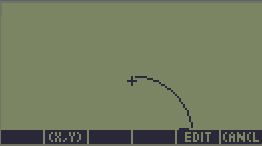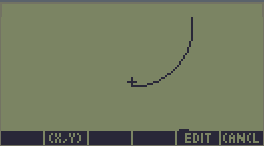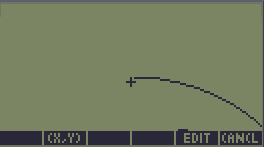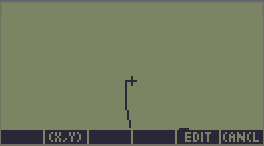Fast Approximative ArcFast Approximative Arc

Note : these versions are superseded by the Fast & Accurate Arc algorithm

This algorithm draws Arcs (partial circles) in the GRAPH PICTure,
using an approximative "circumference length" code.
The result can sometimes be wrong by one pixel.

Input :
5 : X0 (pixel coordinate, 0 is first left column, can be negative)
4 : Y0 (pixel coordinate, 0 is first up line, can be negative)
3 : Radius (pixel, maximum 60)
2 : Start Bearing (degree, 0° is North (up))
1 : End Bearing (degree, 0° is North (up))

Fast Approximative Arc V1.0, for HP48 & HP49/50

Benchmark results, using an HP48SX
(expect later model to perform better) :
Full : 0.50s
Half : 0.29s
Quarter : 0.18s
Eight : 0.12s
1° : 0.07s (Note : 0° means "Full Circle")

Source Code, V1.0

Last edited by Admin on Fri 15 Aug - 3:17; edited 1 time in total

Yann

Number of posts : 174
Registration date : 2008-05-01Cyrille de Brebisson - Very fast Arc algorithm

Introducing an MASD code proposed by Cyrille de Brebisson to draw Arcs.

Cyrille de Brebisson's Arc Toolbox v1.1 : HP48 S/G Binary (1651 Bytes) (Checksum #477Bh)

Input :
6 : Grob ( Optional, will draw in Graphic Area if none provided )
5 : X0
4 : Y0
2 : Start Bearing (°)
1 : End Bearing (°)
Notes :
(0,0) is upper left.
0° is North. Bearing values must be positive.
X0 & Y0 must be positive (Warning : you risk a reset if you don't respect this rule)
All values are limited to 2047 (Grob Width/Height, X, Y & Radius)

This code is extremely fast, as can be shown in following benchmarks :
 Name Input HP48SX HP48GX HP50G 30pixels, Quarter circle X=65,Y=32,R=30,S=90,E=180 30ms 30pixels, Half circle X=65,Y=32,R=30,S=90,E=270 31ms 30pixels, Full circle X=65,Y=32,R=30,S=90,E=90 34ms 60pixels, Full circle X=30,Y=0,R=60,S=90,E=90 56ms 150pixels, 3/4 circle X=65,Y=160,R=150,S=90,E=360 89ms
At the time of this writing, this is the fastest code around.

This code is still based on "pixel counting" approximation,
however this new version has a particularly good algorithm,
resulting in near perfect accuracy as long as R<255.

Above this range, a small inaccuracy of a few pixels (at most) can be witnessed.

30pixels half circle : starts one pixel late, ends correctly100pixels 3/4 circle : starts one pixel late, ends correctlyPrevious Versions :
Cyrille de Brebisson's Arc Toolbox V1.0 : HP48 S/G Binary (1215 Bytes)
with Source Code

Yann